## Coming soon: 3x table, negative numbers and adding decimalsChildren need an awful lot of practice and ‘overlearning’ if they are ever really going to know their tables. By knowing, I mean instant response, so that they know that 3 x 6 is 18 just as quickly as if they had been asked their name! It’s always good to find a slightly different approach and our worksheet next week does just that.

Magic squares come in all sorts of formats and our pages next week are quite challenging as they involve adding negative and positive numbers and are probably most suited to Year 6 children (10/11 yrs old).

We also have a follow up page to an earlier worksheet on adding decimals mentally. The approach is probably the same as adding 2-digit numbers, in that most people seem to do these ‘in their head’ by adding the units first and then adding on the decimals.

## Year 5 Maths Worksheets: Square numbers (3)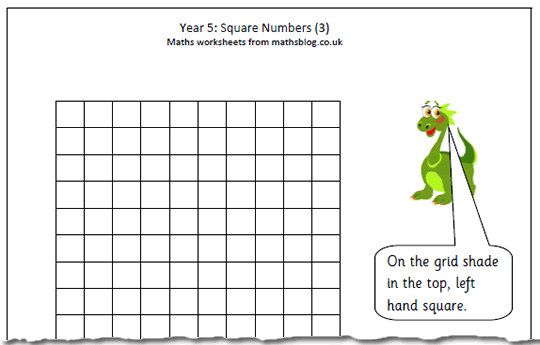‘Maths is all about patterns!’ said my maths lecturer over 30 years ago and this worksheet on square numbers proves just how right he was. It uses a blank 10×10 square to show the pattern made when producing square numbers.

It is interesting to note that the second square number (4) is made by adding the first two odd numbers (1 + 3) and the third square number is made up of the first three odd numbers (1 + 3 + 5 = 9). This pattern continues (for ever!) with the fourth square number (16) made up of (1 + 3 + 5 + 7) and so on. By colouring in the number square it should be apparant to children why this is happening.

Square numbers 3

## Resource of the Week: Year 6 fractions vocabulary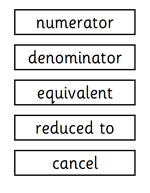Don’t forget that we have a great range of pages which provide information and printable resources on what is expected in each year group by the primary Framework for Maths. This list contains the key vocabulary for Fractions, decimals, percentages and ratio in year 6.
Children will have come across all these words in earlier years, but by the end of year 6 (10/11 yr old) they should have a good understanding of all of them

Fraction   proper fraction     improper fraction

Numerator      denominator

Equivalent        reduced to                 cancel

Half                   quarter                       eighth

Third          sixth                ninth           twelfth

Fifth           tenth                twentieth

Hundredth             thousandth

Proportion            decimal fraction

Decimal point                decimal place

percentage            per cent           %

When spelling, the word twelfth often causes problems. They are all shown in a larger font below if you want to cut them out and print them.

If you are unsure of any of the meanings try one of the free online maths dictionaries such as at
www.mathsphere.co.uk or www.amathsdictionaryforkids.com/
(Taken from Mathematical Vocabulary Book  DfEE)

Year 6 vocabulary: fractions, decimals and percentages

## Year 2 maths worksheet: Addition investigation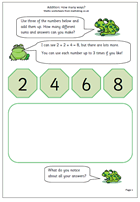Here we have a maths investigation on addition, suitable for year 2. There are four numbers shown. The task is to choose any three numbers and add them up. Each number can be used up to 3 times, but the total sum must not include more than 3 numbers. So the sum could be 2 + 2 + 2, or 2 + 4 + 4 etc.

How many different ways are there of doing this and how many different answers are there.

These mini investigations are all about logical thinking, trying things out, working in a systematic way, recording information and checking that results have not been duplicated. They should also be fun. Most young children will not work in a systematic way, will repeat results and miss results – that is to be expected. But, over time they should begin to develop these skills. This investigation is good at encouraging working in a methodical way, starting with the smallest numbers, or starting with the largest numbers.

Thanks to urbrainy.com for this contribution.

## Times tables: 4 times table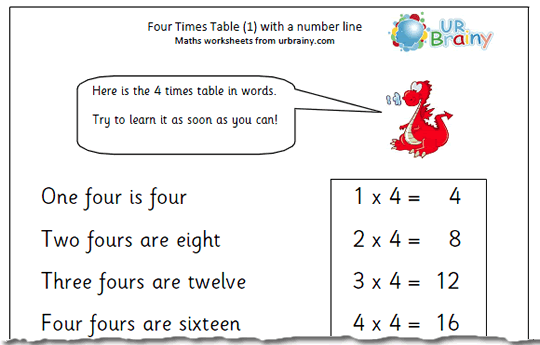The normal route through learning tables is to start with the twos and tens as these are probably the tables most familiar to children. The next stage is usually to learn the fives, followed by fours. The four times table has a number of characteristics which can be pointed out to help children. Firstly, all the answers are even, so must end in 0, 2, 4, 6 or 8. Secondly it is double the two times tables. Doubling is a very powerful mental arithmetic tool and some cultures incorporate it into all their multiplying. Continue reading “Times tables: 4 times table”

## Coming soon: 4x table, addition investigation and square numbers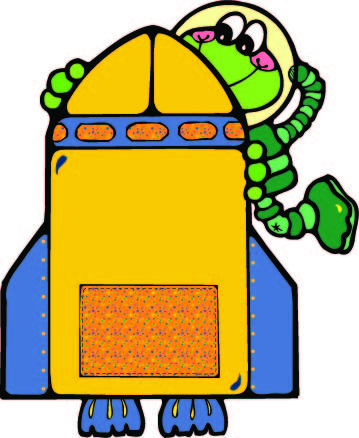Our Four Rules section of the site is developing into a very useful resource for those looking to improve calculating skills. Next week we add to it with a 4x table page. The four times table has a number of characteristics which can be pointed out to help children. Firstly, all the answers are even, so must end in 0, 2, 4, 6 or 8. Secondly it is double the two times tables.

We also have a maths investigation on addition, suitable for year 2. There are four numbers shown. The task is to choose any three numbers and add them up. great for developing a well organised, systematic approach.

Another excellent addition to the site will be the third in our mini series on square numbers, ideal for year 5 children.

## Year 6 maths worksheet: Reading decimal fractions (2)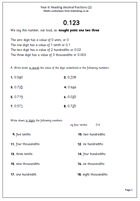This is a follow up page from one published earlier, reinforcing reading decimal fractions.

It is important that children reading decimals correctly. With a decimal such as 0.345 probably the best way to read it is: ‘nought point three, four, five and not nought point three hundred and forty five.

This is also good revision of place value and the use of thousandths. Probably children will come across thousandths only in terms of measurement such as litres and ml, or kilometres and metres.

## Resource of the Week: Booster page for Year 6 SATs.Don’t forget that we have some excellent ‘booster’ pages for children who will be doing their SATs this summer. Here we have some examples of the type of arithmetic questions that come up. An interesting example is the question which asks to put the digits 7, 3 and 4 into the boxes to make the total of 41.Sometimes there is more than one way to do this type of question and it is a matter of using ‘trial and improvement’ to solve it.

One good way to tackle it is to look at the units (in this case 1) and see which two digits added together will put a 1 in the units. The only option here is is to add the digits 7 and 4 (which make 11), put these in the units boxes and it is easy from there on.

Thanks to MathSphere for letting us use this worksheet.

Booster maths worksheet 3

## Maths worksheet: Divide by 10 with remainders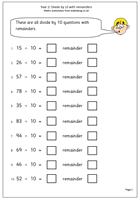This maths worksheet contains a straightforward set of questions on dividing 2-digit numbers by ten, with remainders. It is aimed at children in Year 2 who are just getting to grips with division and know their ten times table.

The best way to do these is to go through the ten times table until the nearest whole ten below the number is found, then add on to reach the remainder. When dividing by ten the remainder can never be greater than ten.

Further division worksheets can be found both in the Four Rules section under Division or in the separate year groups under Calculating.

Divide by 10 with remainders

## Times tables: More 3x tables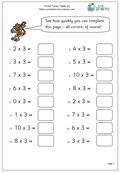This is a slightly harder worksheet than the last one published on the three times table. This page does not have a number line to help and the questions are asked in different order eg 6 x 3 or 3 x 6. This could be used as a timed challenge for those who are gaining confidence in learning the table.

Interestingly, when I whizzed through this page mentally I suddenly realised that I did not necessarily work out the answer in the order shown on the page. For example I thought of 2 x 3 as 2 x 3 = 6, but when it cam to 3 x 5 I thought of it as 5 x 3 = 15. I saw the two numbers which needed multiplying and automatically chose the easier way to do them. However, this would not be the case for children who do not know all their tables.

3 times table (2)